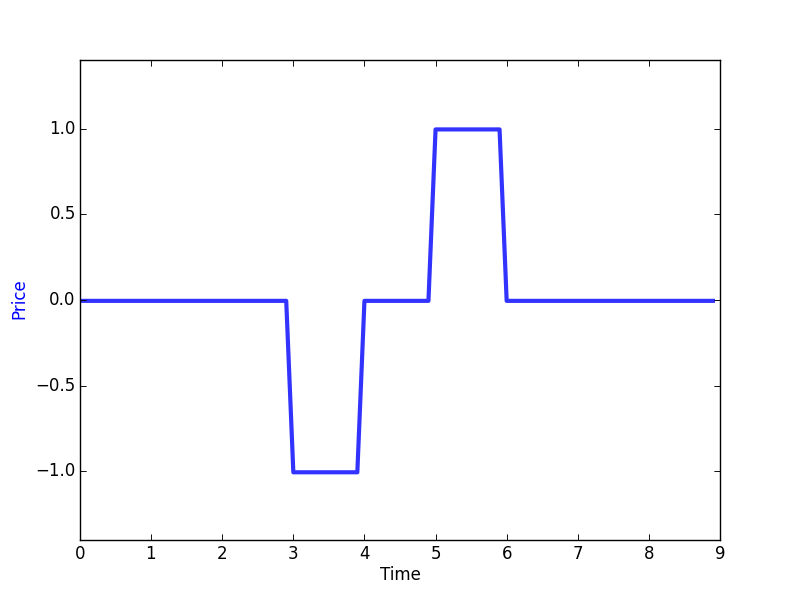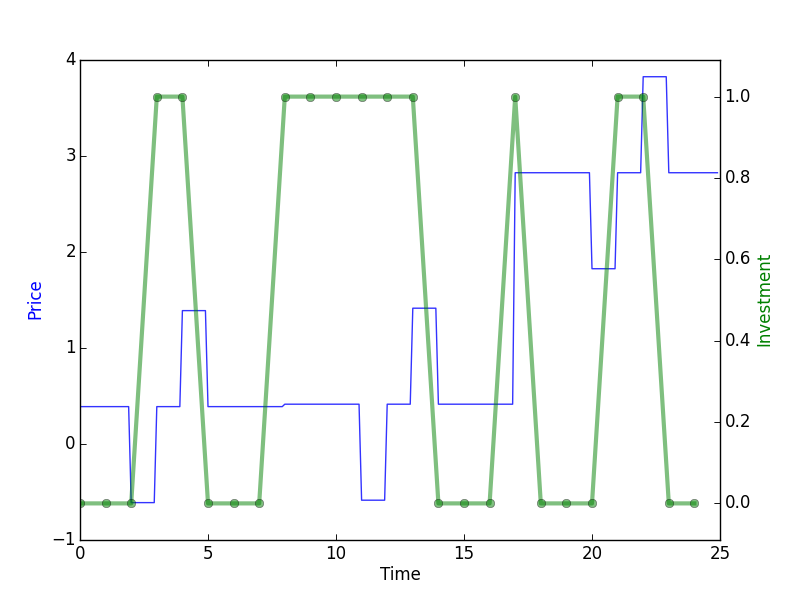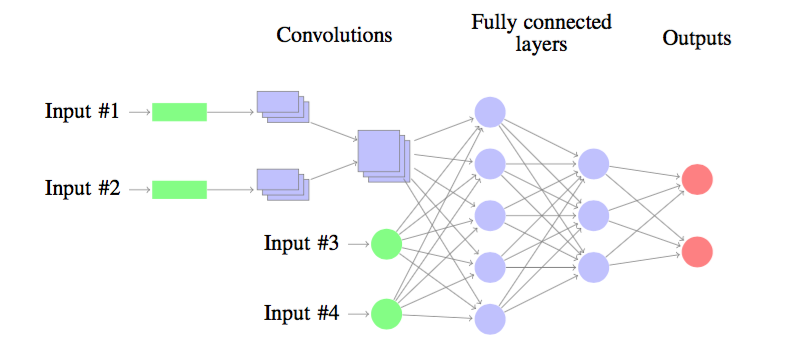# Toy environment with time series¶

## Description of the environement¶

This environment simulates the possibility of buying or selling a good. The agent can either have one unit or zero unit of that good. At each transaction with the market, the agent obtains a reward equivalent to the price of the good when selling it and the opposite when buying. In addition, a penalty of 0.5 (negative reward) is added for each transaction.

The price pattern is made by repeating the following signal plus a random constant between 0 and 3:You can see how this environement is built by looking into the file Toy_env.py in examples/toy_env/. It is important to note that any environment derives from the base class Environment and you can refer to it in order to understand the required methods and their usage.

## How to run¶

A minimalist way of running this example can be found in the file run_toy_env_simple.py in examples/toy_env/.

• First, we need to import the agent, the Q-network, the environement and some controllers
 1 2 3 4 5 6  from deer.agent import NeuralAgent from deer.learning_algos.q_net_keras import MyQNetwork from Toy_env import MyEnv as Toy_env import deer.experiment.base_controllers as bc 
• Then we instantiate the different elements as follows:
  1 2 3 4 5 6 7 8 9 10 11 12 13 14 15 16 17 18 19 20 21 22 23 24 25 26 27 28 29 30 31 rng = np.random.RandomState(123456) # --- Instantiate environment --- env = Toy_env(rng) # --- Instantiate qnetwork --- qnetwork = MyQNetwork( environment=env, random_state=rng) # --- Instantiate agent --- agent = NeuralAgent( env, qnetwork, random_state=rng) # --- Bind controllers to the agent --- # Before every training epoch, we want to print a summary of the agent's epsilon, discount and # learning rate as well as the training epoch number. agent.attach(bc.VerboseController()) # During training epochs, we want to train the agent after every action it takes. # Plus, we also want to display after each training episode (!= than after every training) the average bellman # residual and the average of the V values obtained during the last episode. agent.attach(bc.TrainerController()) # We also want to interleave a "test epoch" between each training epoch. agent.attach(bc.InterleavedTestEpochController(epoch_length=500)) # --- Run the experiment --- agent.run(n_epochs=100, epoch_length=1000) 

## Results¶

Navigate to the folder examples/toy_env/ in a terminal window. The example can then be run by using

python run_toy_env_simple.py


You can also choose the full version of the launcher that specifies the hyperparameters for better performance.

python run_toy_env.py


Every 10 epochs, a graph is saved in the ‘toy_env’ folder. You can then visualize the test policy at the end of the training:In this graph, you can see that the agent has successfully learned to take advantage of the price pattern to buy when it is low and to sell when it is high. This example is of course easy due to the fact that the patterns are very systematic which allows the agent to successfully learn it. It is important to note that the results shown are made on a validation set that is different from the training and we can see that learning generalizes well. For instance, the action of buying at time step 7 and 16 is the expected result because in average this will allow to make profit since the agent has no information on the future.

## Using Convolutions VS LSTM’s¶

So far, the neural network was build by using a convolutional architecture as follows:The neural nework processes time series thanks to a set of convolutions layers. The output of the convolutions as well as the other inputs are followed by fully connected layers and the ouput layer.

When working with deep reinforcement learning, it is also possible to work with LSTM’s (see for instance this introduction to LSTM's)

If you want to use LSTM’s architecture, you can import the following libraries

from deer.learning_algos.NN_keras_LSTM import NN as NN_keras


and then instanciate the qnetwork by specifying the ‘neural_network’ as follows:

qnetwork = MyQNetwork(
env,
neural_network=NN_keras)